﻿ PipeLay > Software Components & Operation > Analysis & Postprocessing > Postprocessing Subcomponents > S-N Curve > S-N Curve Data Inputs

# S-N Curve Data Inputs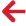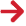Note

This component is not available in PipeLay Starter Edition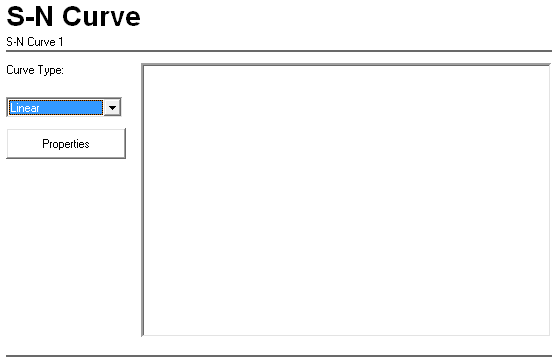Input Description Curve Type: This drop-down list is used to select the format in which the S-N curve is defined. The options are Linear (the default), Piecewise Linear and Data Pairs. Properties Click on this button to display a dialog that allows you to enter the properties of the S-N Curve component. The dialog that is displayed depends on the option that you selected in the Curve Type drop-down list. See the ‘S-N Curve – Linear Dialog’, ‘S-N Curve – Piecewise Linear Dialog’ or ‘S-N Curve – Data Pairs Dialog’ section, as appropriate, for more information.

## S-N Curve Linear Dialog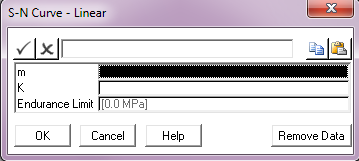Input Description m: The first parameter defining the S-N curve. S-N curves are generally defined in the form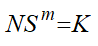where S denotes stress range, N the number of cycles to failure at this range, and m and K are constants. Taking logarithms of both sides and rearranging gives: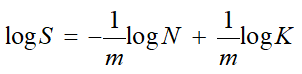which is the equation of a straight line when log S is plotted against log N. In this case m is the inverse slope and K is a function of the line intercept K: The second parameter defining the S-N curve. See the description of the m input above for more information. Units: [(MPa)m] or [(ksi)m] Endurance Limit: A stress range value below which no fatigue damage occurs, regardless of the number of cycles. The specification of an Endurance Limit is optional. If omitted, the value defaults to 0, which means that there is no endurance limit. Units: [MPa] or [ksi]

## S-N Curve – Piecewise Linear Dialog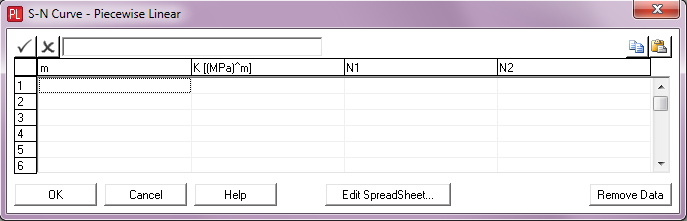A piecewise-linear S-N curve is one which plots as a series of straight line segments when log S is plotted against log N. In this case m and K vary between line segments. The N1 and N2 values specify the segment of the S-N curve (number of cycles to failure) axis where each m and K combination apply.

 Input Description m: The first parameter defining the S-N curve. K: The second parameter defining the S-N curve. Units: [(MPa)m] or [(ksi)m]

## S-N Curve – Data Pairs Dialog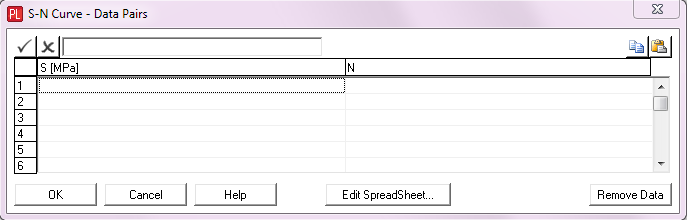This dialog allows you to specify an S-N curve directly.  Use as many lines as you need to completely define your S-N curve

 Input Description S: A stress range value. Units: [MPa] or [ksi] N: The number of cycles to cause failure at this stress range.